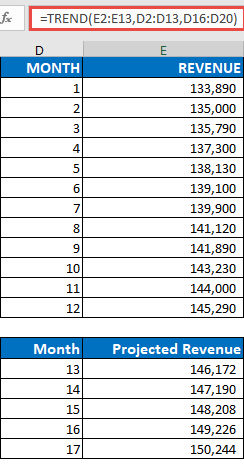## Excel Office

Excel How Tos, Tutorials, Tips & Tricks, Shortcuts

# TREND function: Description, Usage, Syntax, Examples and Explanation

## What is TREND function in Excel?

TREND function in Excel is a Statistical Function that computes the linear trend line based on the given linear set of data. TREND in Excel calculates the predictive values of Y for given array values of X and uses the least square method based on the given two data series.

Worked Example:   Forecast vs Trend Function in Excel

## Explanation of TREND function

TREND returns values along a linear trend. Fits a straight line (using the method of least squares) to the arrays known_y’s and known_x’s. Returns the y-values along that line for the array of new_x’s that you specify.Note: If you have a current version of Office 365, then you can input the formula in the top-left-cell of the output range (cell E16 in this example), then press ENTER to confirm the formula as a dynamic array formula. Otherwise, the formula must be entered as a legacy array formula by first selecting the output range (E16:E20), input the formula in the top-left-cell of the output range (E16), then press CTRL+SHIFT+ENTER to confirm it. Excel inserts curly brackets at the beginning and end of the formula for you.
Click link to see practical illustration of TREND functionTREND function.
Worked Example:   Forecast vs Trend Function in Excel
Worked Example:   Forecast vs Trend Function in Excel